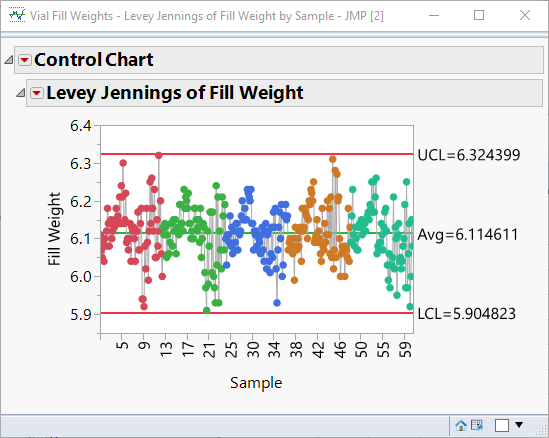Choose Language Hide Translation Bar

## Getting calculated control limits from a Levey Jennings chart

Hi Everyone,

I'm currently working on a script that generates a LJ chart.  I need to drop a bunch of lines in there and must make sure the scale of the Y-axis allows to see all these lines.

This all works perfectly except for the calculated control limits.  Is there any way that I can get these calculated limits, preferably before I generated the chart, so I can use them in the calculation I make to determine the Y-axis scale?

Thanks!

--Jan

1 ACCEPTED SOLUTION

Accepted Solutions
Highlighted

## Re: Getting calculated control limits from a Levey Jennings chart

Levey Jennings just uses the long term sigma to compute control limits, so you can just take the standard deviation of the entire column of the response you are plotting.  Here's an example using the Vial Fill Weights example from the Sample Data Library.

``````dt = Data Table("Vial Fill Weights");
y = :Fill Weight << Get Values;
y_bar = mean(y);
sigma = std dev(y);

UCL = y_bar + 3*sigma;
LCL = y_bar - 3*sigma;``````

This returns 5.90482338181099 and 6.32439884041124 for LCL and UCL respectively.  This matches the Levey Jennings limits:-- Cameron Willden
Highlighted

## Re: Getting calculated control limits from a Levey Jennings chart

Levey Jennings just uses the long term sigma to compute control limits, so you can just take the standard deviation of the entire column of the response you are plotting.  Here's an example using the Vial Fill Weights example from the Sample Data Library.

``````dt = Data Table("Vial Fill Weights");
y = :Fill Weight << Get Values;
y_bar = mean(y);
sigma = std dev(y);

UCL = y_bar + 3*sigma;
LCL = y_bar - 3*sigma;``````

This returns 5.90482338181099 and 6.32439884041124 for LCL and UCL respectively.  This matches the Levey Jennings limits:-- Cameron Willden
Article Labels

There are no labels assigned to this post.